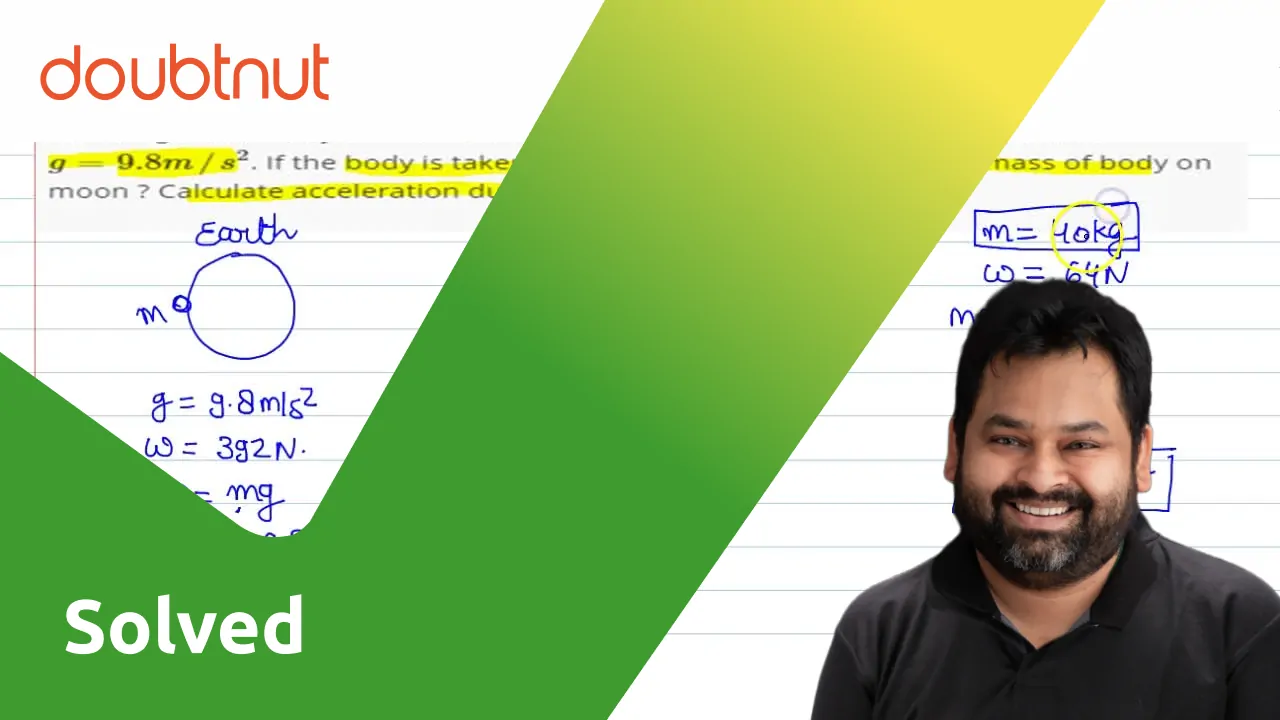Doubtnut App - Get 50 Lakh+ video solutions better than GoogleInstall Now

# The weight of a body on the surface of earth is 392N. What is its mass, when g=9.8m/s2. If the body is taken to moon, it weighs 64 N. what is mass of body on moon ? Calculate acceleration due to gravity on moon.

Text Solution
Verified by Experts

## From We=mge m=Wege=3929.2=40 kg. mass on moon=mass on earth =40kg Again, from Wm=mgm gm=Wmm=6440=1.6m/s2